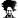# Merge All worksheet from multiple workbooks to one workbook

#### anshul.malhotra

##### Member
Hi All,

I am looking for help as I am not a pro in VBA but have googled many code and fix my task, however this it is not helping me;

I want to merge 184 excel workbook, where each workbook has 3-5 worksheet, with Workbook name stamp in last column and Sheet tab name in next column following workbook name. But the below code is working on only one worksheet not all and also I am not able to add worksheet name from where the data has been copied from.

below is the code I am using

Code:
``````Sub Merge_with_Col_added()
Dim fn, ws As Worksheet, e, flg As Boolean, LastR As Range, wsName As String
fn = Application.GetOpenFilename("Excel(*.xls*),*.xls*", MultiSelect:=True)
If Not IsArray(fn) Then Exit Sub
ws.Name = "Combined"
Set ws = ActiveWorkbook.Sheets("Combined")
Application.ScreenUpdating = False
ws.Cells.Clear
For Each e In fn
With Workbooks.Open(e)
With .Sheets(1)
wsName = .Name
If Not flg Then
.Rows(1).Copy ws.Cells(1)
ws.Columns(1).Insert
ws.Cells(1).Value = "Sheet name"
flg = True
End If
Set LastR = ws.Cells(Rows.Count, 2).End(xlUp)(2)
With .Range("a1").CurrentRegion
With .Resize(.Rows.Count - 1).Offset(1)
.Copy LastR
LastR(, 0).Resize(.Rows.Count).Value = CreateObject("Scripting.FileSystemObject").GetBasename(e)
End With
End With
End With
.Close False
End With
Next
ws.Range("a1").CurrentRegion.Columns.AutoFit
Application.ScreenUpdating = True
Set ws = Nothing
End Sub``````

Anshul

Last edited by a moderator:

#### p45cal

##### Well-Known Member
First, for dealing with all sheets in each file, try:
Code:
``````Sub Merge_with_Col_added()
Dim fn, ws As Worksheet, e, flg As Boolean, LastR As Range, wsName As String
fn = Application.GetOpenFilename("Excel(*.xls*),*.xls*", MultiSelect:=True)
If Not IsArray(fn) Then Exit Sub
ws.Name = "Combined"
Set ws = ActiveWorkbook.Sheets("Combined")
Application.ScreenUpdating = False
ws.Cells.Clear
For Each e In fn
With Workbooks.Open(e)
For Each sht In .Sheets
With sht
wsName = .Name
If Not flg Then
.Rows(1).Copy ws.Cells(1)
ws.Columns(1).Insert
ws.Cells(1).Value = "Sheet name"
flg = True
End If
Set LastR = ws.Cells(Rows.Count, 2).End(xlUp)(2)
With .Range("a1").CurrentRegion
With .Resize(.Rows.Count - 1).Offset(1)
.Copy LastR
LastR(, 0).Resize(.Rows.Count).Value = CreateObject("Scripting.FileSystemObject").GetBasename(e)
End With    '.Resize(.Rows.Count - 1).Offset(1)
End With    '.Range("a1").CurrentRegion
End With    'sht
Next sht
.Close False
End With    'Workbooks.Open(e)
Next    'e
ws.Range("a1").CurrentRegion.Columns.AutoFit
Application.ScreenUpdating = True
Set ws = Nothing
End Sub``````

#### anshul.malhotra

##### Member
First, for dealing with all sheets in each file, try:
Code:
``````Sub Merge_with_Col_added()
Dim fn, ws As Worksheet, e, flg As Boolean, LastR As Range, wsName As String
fn = Application.GetOpenFilename("Excel(*.xls*),*.xls*", MultiSelect:=True)
If Not IsArray(fn) Then Exit Sub
ws.Name = "Combined"
Set ws = ActiveWorkbook.Sheets("Combined")
Application.ScreenUpdating = False
ws.Cells.Clear
For Each e In fn
With Workbooks.Open(e)
For Each sht In .Sheets
With sht
wsName = .Name
If Not flg Then
.Rows(1).Copy ws.Cells(1)
ws.Columns(1).Insert
ws.Cells(1).Value = "Sheet name"
flg = True
End If
Set LastR = ws.Cells(Rows.Count, 2).End(xlUp)(2)
With .Range("a1").CurrentRegion
With .Resize(.Rows.Count - 1).Offset(1)
.Copy LastR
LastR(, 0).Resize(.Rows.Count).Value = CreateObject("Scripting.FileSystemObject").GetBasename(e)
End With    '.Resize(.Rows.Count - 1).Offset(1)
End With    '.Range("a1").CurrentRegion
End With    'sht
Next sht
.Close False
End With    'Workbooks.Open(e)
Next    'e
ws.Range("a1").CurrentRegion.Columns.AutoFit
Application.ScreenUpdating = True
Set ws = Nothing
End Sub``````
Thanks p45cal... this work for multiple sheet tabs now, can you help me getting the sheet tab name too next to workbook name in column B

#### p45cal

##### Well-Known Member
Code:
``````Sub Merge_with_Col_added()
Dim fn, ws As Worksheet, e, flg As Boolean, LastR As Range, wsName As String
fn = Application.GetOpenFilename("Excel(*.xls*),*.xls*", MultiSelect:=True)
If Not IsArray(fn) Then Exit Sub
ws.Name = "Combined"
Set ws = ActiveWorkbook.Sheets("Combined")
Application.ScreenUpdating = False
ws.Cells.Clear
For Each e In fn
With Workbooks.Open(e)
For Each sht In .Sheets
With sht
wsName = .Name
If Not flg Then
.Rows(1).Copy ws.Cells(1)
ws.Columns(1).Resize(, 2).Insert
ws.Cells(1, 1).Value = "Workbook"
ws.Cells(1, 2).Value = "Sheet name"
flg = True
End If
Set LastR = ws.Cells(Rows.Count, 1).End(xlUp).Offset(1, 2)
Application.Goto LastR
With .Range("a1").CurrentRegion
With .Resize(.Rows.Count - 1).Offset(1)
.Copy LastR
'LastR(, 0).Resize(.Rows.Count).Value = CreateObject("Scripting.FileSystemObject").GetBasename(e)
LastR.Offset(, -2).Resize(.Rows.Count).Value = CreateObject("Scripting.FileSystemObject").GetBasename(e)
LastR.Offset(, -1).Resize(.Rows.Count).Value = sht.Name
End With    '.Resize(.Rows.Count - 1).Offset(1)
End With    '.Range("a1").CurrentRegion
End With    'sht
Next sht
.Close False
End With    'Workbooks.Open(e)
Next    'e
ws.Range("a1").CurrentRegion.Columns.AutoFit
Application.ScreenUpdating = True
Set ws = Nothing
End Sub``````

•anshul.malhotra

#### anshul.malhotra

##### Member
T
Code:
``````Sub Merge_with_Col_added()
Dim fn, ws As Worksheet, e, flg As Boolean, LastR As Range, wsName As String
fn = Application.GetOpenFilename("Excel(*.xls*),*.xls*", MultiSelect:=True)
If Not IsArray(fn) Then Exit Sub
ws.Name = "Combined"
Set ws = ActiveWorkbook.Sheets("Combined")
Application.ScreenUpdating = False
ws.Cells.Clear
For Each e In fn
With Workbooks.Open(e)
For Each sht In .Sheets
With sht
wsName = .Name
If Not flg Then
.Rows(1).Copy ws.Cells(1)
ws.Columns(1).Resize(, 2).Insert
ws.Cells(1, 1).Value = "Workbook"
ws.Cells(1, 2).Value = "Sheet name"
flg = True
End If
Set LastR = ws.Cells(Rows.Count, 1).End(xlUp).Offset(1, 2)
Application.Goto LastR
With .Range("a1").CurrentRegion
With .Resize(.Rows.Count - 1).Offset(1)
.Copy LastR
'LastR(, 0).Resize(.Rows.Count).Value = CreateObject("Scripting.FileSystemObject").GetBasename(e)
LastR.Offset(, -2).Resize(.Rows.Count).Value = CreateObject("Scripting.FileSystemObject").GetBasename(e)
LastR.Offset(, -1).Resize(.Rows.Count).Value = sht.Name
End With    '.Resize(.Rows.Count - 1).Offset(1)
End With    '.Range("a1").CurrentRegion
End With    'sht
Next sht
.Close False
End With    'Workbooks.Open(e)
Next    'e
ws.Range("a1").CurrentRegion.Columns.AutoFit
Application.ScreenUpdating = True
Set ws = Nothing
End Sub``````

Thanks a lot for your help...

#### anshul.malhotra

##### Member